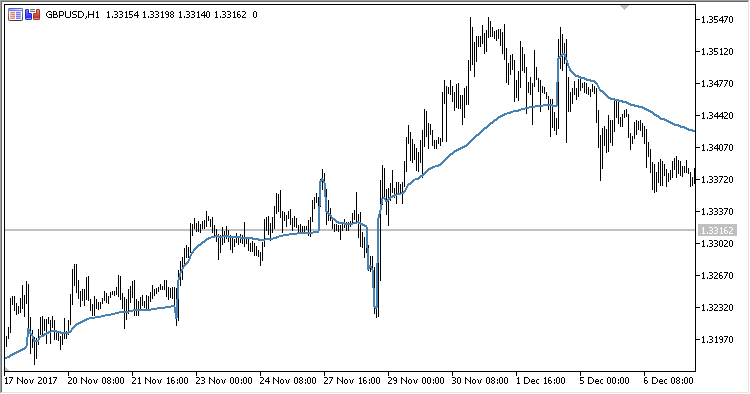Interesting script?
So post a link to it -
let others appraise it

You liked the script? Try it in the MetaTrader 5 terminal# VolatilityMA - indicator for MetaTrader 5

Views:
952
Rating:
Published:
2018.07.09 11:06

The indicator represents a moving average that resets its calculation period, as soon as the current standard deviation exceeds the previous one twice or more when the bar is closing.

It is useful for detecting the trade direction.

• Period - calculation period
• Barrier - if this barrier is exceeded, the period calculation is reset to the minimum
• Applied price - calculation price
```VolMA = SMA(Price, Length)
```

where:

```Price = Applied price
PrevPrice = previous Applied price
If Abs(sDevNow) > Barrier
Length = 1
otherwise
Length = PrevLength+1
sDevNow = RAW / Price * sDev
sDev = SQRT(VAR / Period)

VAR = POW(RAW[Period] + RawAVG
RAW = (Price - PrevPrice) / Price
RawAVG = SMA(RAW, Period)

```Translated from Russian by MetaQuotes Software Corp.
Original code: https://www.mql5.com/ru/code/20994TCI

Indicator Trend Confirmation IndexSpeed_of_tradeWAMICADX